Courses

# Test: Stereochemistry Level - 2

## 30 Questions MCQ Test Organic Chemistry | Test: Stereochemistry Level - 2

Description
This mock test of Test: Stereochemistry Level - 2 for Chemistry helps you for every Chemistry entrance exam. This contains 30 Multiple Choice Questions for Chemistry Test: Stereochemistry Level - 2 (mcq) to study with solutions a complete question bank. The solved questions answers in this Test: Stereochemistry Level - 2 quiz give you a good mix of easy questions and tough questions. Chemistry students definitely take this Test: Stereochemistry Level - 2 exercise for a better result in the exam. You can find other Test: Stereochemistry Level - 2 extra questions, long questions & short questions for Chemistry on EduRev as well by searching above.
QUESTION: 1

Solution:
QUESTION: 2

### The compound in ‘R’ configuration is:

Solution:

In option B and C one of the terminal carbons has the same group, so it is neglected and in A H has least priority. Rotation gives S configuration and since it is at wedge position so we reverse it and S -> R we get the configuration to be R and hence A is correct.

QUESTION: 3

### Draw the structure of (2R, 3S) – 2, 3 – dichloropentane:

Solution:
QUESTION: 4

The most stable confirmation of following compound is: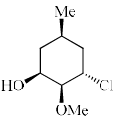Solution:
QUESTION: 5

Structure of 1, 2-dimethylcyclohexane is show below. Which of the following is an enantiomer to the given conformer: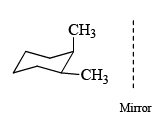Solution: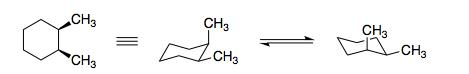QUESTION: 6

Which is the most stable conformation of 1-chloro-4-methylcyclohexane:

Solution:
QUESTION: 7

Which of the following is a structure representation is R-Lactic acid: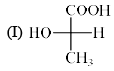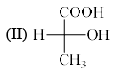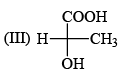Solution:

(R)-lactic acid is an optically active form of lactic acid having (R)-configuration. It has a role as an Escherichia coli metabolite and a human metabolite. It is a conjugate acid of a (R)-lactate. It is an enantiomer of a (S)-lactic acid.

The chiral carbon atom has all four different group attached to it.

∴ It has one asymmetric or chiral carbon atom.

There option b) is correct.

QUESTION: 8

The favored conformation (gauche, anti) for the molecule 1, 2-dichloro ethane; and 1,2-ethanediol will be respectively:

Solution:
QUESTION: 9

Which of the following term best describes the pair of compound shown below: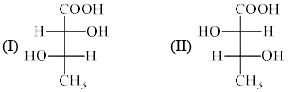Solution:
QUESTION: 10

The major product is formed in the following reaction is: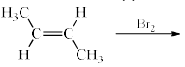Solution:
QUESTION: 11

The configurations at the three chiral centres in the bicyclodecano l given below, are: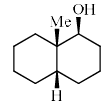Solution:
QUESTION: 12

The S enantiomer of ibuprofen is responsible for its pain – relieving properties. Which one of the following structures shown in (S) – ibuprofen:

Solution:

Giving the numbers according to their priority we get: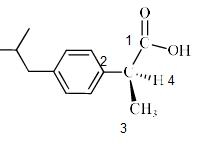Since 1 -> 2 -> 3 -> 4 is in the anti clockwise direction, It has S configuration.

Hence D is correct.

QUESTION: 13

The major product formed in the following reaction is: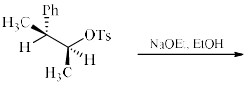Solution:

Here, basic conditions are given so

1. Most acidic H+ ion is removed which is attached to the carbon atom at benzylic position.

2. Then to stabilize the carbanion, elimination reaction mechanism takes place.

3. Leading to the removal of leaving group -OTs, forming a double bond between the two carbon atoms.

Hence D is correct.

QUESTION: 14

Draw the structure of (1 R, 2 S, 3 S)-1, 2-dibromo-3-ethyl cyclo hexane:

Solution:
QUESTION: 15

The absolute configurations of the chiral centers in X and Y are: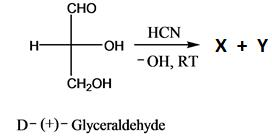Solution: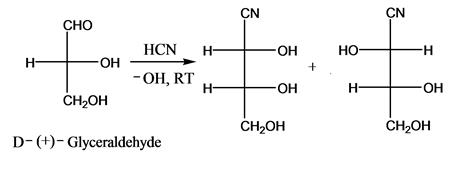QUESTION: 16

Stereoisomers which are not mirror image isomers are:

Solution:
QUESTION: 17

Racemisat ion process is:

Solution:
QUESTION: 18

The configuration of the following position of (I) and (II) is: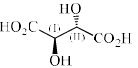Solution:
QUESTION: 19

Resolution is called as:

Solution:
QUESTION: 20

Which is the correct structure of D-Glyceraldehyde:

Solution:

All the options A, B and C are same

1. When A is rotated by 90 degrees two times i.e. 180 degrees in plane of your screen then compound C will be obtained

2. Now by switching A two times or ‘even’ switch compound B will be obtained and configuration remained same as the switch was even

Hence all are the same, so, D is correct.

QUESTION: 21

Which of the following structure represents meso compounds:

Solution:
QUESTION: 22

The two compounds shown below are: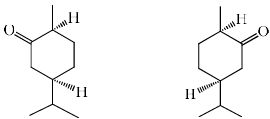Solution:
QUESTION: 23

Which of the following statements is true for the compound (R)-2-butanol:

Solution:
QUESTION: 24

What kind of isomers are the two compound below: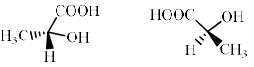Solution:
QUESTION: 25

Among the structures given below, the most stable conformation for the following compound is: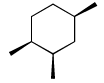Solution:

Explanation :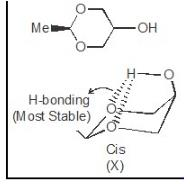QUESTION: 26

Which of the following term correctly describes the structural relationship between cis-1,3 dimethyl cyclopentane and trans-1,3-dimethyl cyclopentane:

Solution:
QUESTION: 27

The gauche conformation (θ = 60o) of n-butane possess:

Solution:
QUESTION: 28

The absolute configuration of the two stereogenic (Chiral) centers in the following molecule is: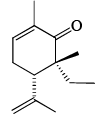Solution:

If minimum priority group on horizontal line is

Clockwise direction ⇒S

Anticlockwise rotation ⇒R

5S, 6R

QUESTION: 29

The gauche interaction values for Me/Me, Me/Br and Br/Br are 3.3, 0.8 and 3.0 kJ/mol, respectively. Among the following, the most stable conformation of 2,3-dibromobutane is:

Solution:
QUESTION: 30

The most stable conformations of 1,2-difluoroethane and dl-2, 3-butanediol are:

Solution:

The -F and -OH groups in gauche positions to each other help them get involved in dipole-dipole interactions and h-bond interactions respectively. Gauche position is optimum so as to provide proximity for the stabilising interaction but not too close as in eclipsed positions to give rise to steric strains.